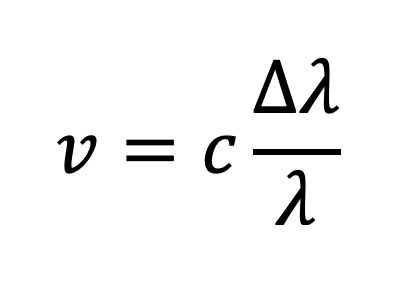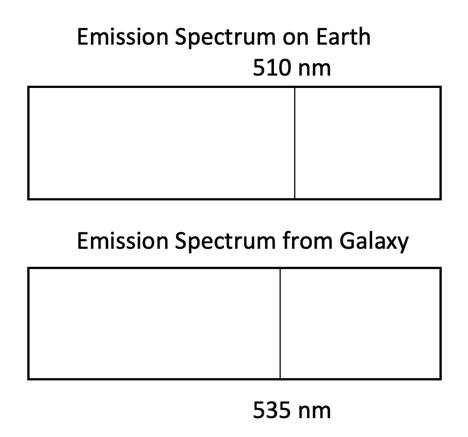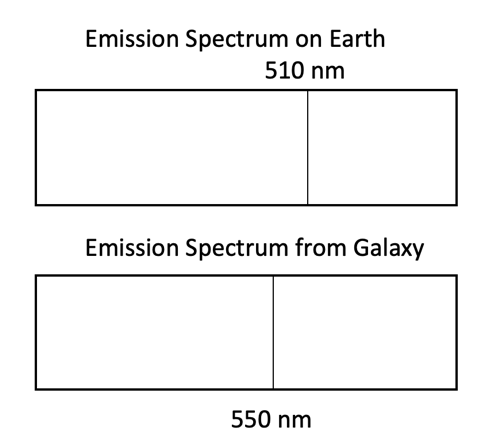# HSC Physics Questions You Should Prepare For (IN-DEPTH)

With several changes present in the new HSC Physics curriculum, students must not only be mindful of them but also learn how to use them to their advantage and to maximise their marks.

### Circular Motion and Torque

While Module 5: Advanced Mechanics contains content from the old Space module, it explores two key concepts in detail - circular motion and torque.

• Students are advised to spend more time understanding circular motion principles (including work done during a uniform circular motion) and more importantly calculations involving circular motion.
• Students are advised to practise explaining the definition of torque and performing calculations in the context of advanced mechanics and motors and generators.

Question 1: A 25 N force acts on a 1-metre long lever arm that has a mass of 5 kg. If the force is always orthogonal to the lever arm, calculate how many revolutions (to nearest 2 decimal places) it would take for the arm to reach an angular velocity of 35 rad/s. (3 marks)

Is there work done on the lever arm during this process? Explain your answer. (1 mark)

Question 2: Define torque (1 mark)

### Energy Changes in Space

The new curriculum has a focus on mathematical derivation, especially of equations in Module 5: Advanced Mechanics.

• Students are advised to fully understand the changes in an object's kinetic and gravitational energy during its launch into space or transition between altitudes.
• Students are advised to understand and memorise the derivation process of several equations. Remember that the total energy of an object in a stable orbit is exactly half of its gravitational potential energy.

Question 1: Explain the energy changes that occur to a satellite during its ascension into space from the surface of Earth. (4 marks)

Question 2: Quantitatively show that the total energy of an object in a stable orbit is exactly half of its gravitational potential energy. (3 marks)

### Applications of Electromagnetic Induction

Module 6: Electromagnetism, out of the 4 modules in HSC Physics, is arguably the module that has experienced the fewest changes.

Emphasis on social and environmental impact has been replaced with deeper understanding of underlying physics principles and associated applications.

Question 1:

A truck driver (of a truck which uses inductive braking) activates the brakes before colliding with a vehicle in front of the truck. The truck’s speed (shown on the speedometer) decreases from 100 km/hr to 40 km/hr within the first second then 40 km/hr to a complete stop in the next second.

Explain how inductive braking works and why the truck decelerates more in the first second than the second. (3 marks)

Question 2: Compare the physics principles behind the function of DC motors and DC generators. (4 marks)

### James' Maxwell's Contribution to Electromagnetism

Maxwell's contribution to classical physics is new to the HSC Physics curriculum. As such, a focus on and in-depth understanding of this part of the syllabus is recommended.

• Students are advised to clearly understand the main contributions by Maxwell including unification of electric and magnetic fields, prediction of electromagnetic waves and the speed of EM waves.

Question 1:

(a) Describe Hertz’s production of radiowaves with the aid of a diagram. (2 marks)

(b) Explain how Hertz managed to calculate the speed of radiowaves. (2 marks)

(c) Explain how Hertz’s production radiowaves validates Maxwell’s theory regarding electromagnetic waves. (2 marks)

### Diffraction

The Nature of Light would never be complete without the exploration of light's diffractive properties. This new inclusion in the HSC Physics curriculum entails a wide range of questions (theory and calculation) as well as a steep learning curve (in other words, deeper understanding of diffraction would likely yield more advantage during trials and the HSC exam).

• Students are advised to understand why light diffracts using Huygens' circular wavelet model.
• Students are advised to practise performing calculation questions using various equations and trigonometric ratios.Question 1: The diagram below shows a double-slit set-up and the resultant light intensity pattern. The incident light has a wavelength of 560 nm.Determine the distance of separation/spacing between the two slits. (2 marks)

### Relationship Between Experimental Evidence and Scientific Models

This applies to the entire HSC Physics course but primarily concerns Module 7: The Nature of Light and Module 8: From The Universe to The Atom

When studying for scientific models, we encourage students to identify and appreciate experiments that are related to these models, and more importantly how they lead to the models' validation and acceptance.

Question 1: Evaluate the importance of experimental evidence in the development of the wave model of light. (6 marks)

Question 2: Describe an experiment that supports Einsteins' theory of special relativity. (3 marks)

Question 3: With reference to TWO experiments, analyse the important relationship between experimental evidence and validation of scientific models. (6 marks)

### Doppler's Effect and Hubble's Theory

This is again a new addition to the HSC Physics curriculum (previously only in preliminary physics and Optional Module: Astrophysics) and therefore we recommend greater emphasis during preparation.

• Students are advised to revise Doppler's Effect from Year 11 Physics and use equations to perform calculations in the context of receding galaxies relative to Earth:Question 1:

(a) Calculate the velocity of a particular galaxy relative to Earth using the two emission spectra below.(b) The distance between this galaxy and the Milky Way is 2.5 x 1012 Find the Hubble’s constant.

The emission spectrum of the same galaxy is captured 5000 years later as shown below:(c) Assuming Hubble’s constant does not change with time, calculate the new distance between this galaxy and the Milky Way.

### J.J. Thomson's and Robert Millikan's Experiments

As alluded to before, the new HSC Physics curriculum has a greater emphasis on experiments and their importance in scientific advancement.

We believe that Thomson's and Millikan's experiments are not only important to remember and know how to describe, their impacts and contribution to the atomic model are equally as important.

• Students are advised to recognise and appreciate the aim of Thomson's experiment (to determine the charge to mass ratio of cathode rays) as well as the significance of its results (proved the particle nature of cathode rays, confirmed its identity to be a stream of electrons and showed that an electron's mass is ~1800 times smaller than a proton's).
• Students are advised to recognise and appreciate the aim of Millikan's experiment (to determine the charge of an electron) as well as the significance of its results (proved that the charge of electrons is quantised).
• Students are advised to practise calculation questions involving Millikan's oil-drop experiment.
• Students are advised to recognise and appreciate the inherent similarity between the two experiments. Thomson and Millikan demonstrated that it is possible to manipulate sub-atomic particles to such a precise degree, allowing them to obtain meaningful results.

Question 1: Evaluate the importance of J.J. Thomson’s experiment involving cathode rays. (6 marks)

Question 2: Describe Millikan's oil drop experiment and explain how he was able to determine the charge of an electron (5 marks)

Question 3: In a version of Millikan’s oil-drop experiment, an oil drop with mass 2.6 x 10-11 g falls with an acceleration of 1.2 ms-2 in the direction of gravity while the electric field is turned on. The potential difference across the electric field is 7.0 x 104 V and the distance of plate separation is 25 cm. Assume drag force is negligible. How many excess electrons are there in this particular oil droplet?

### de Broglie and Schrödinger

de Broglie's matter wave and particle-wave duality concepts were part of the old Optional Module: Quantum to Quarks.

However, the new curriculum explores the concepts in more depth with the inclusion of Schrödinger's probabilistic model of the atom.

• Students are advised to spend more time researching and understanding the concepts and the relationship between de Broglie's and Schrödinger's work (the limitation of de Broglie's wave model of the atom gave rise to the probabilistic model).
• Students are advised to understand the probabilistic model in more detail as well as learning the concept of orbitals.

Question 1: Explain how de Broglie's matter wave hypothesis was validated by experimental evidence and why it overcomes limitations of Bohr's atomic model. (6 marks)

Question 2: Assess the contribution from Schrödinger to the development of the atomic model. (5 marks)

Still want more questions to practise? View our collection of HSC Physics mock exam papers (new NSW curriculum)!